• 对于参数估计，统计学界的两个学派分别提供了不同的解决方案： 频率主义学派（Frequentist）认为参数虽然未知，但却是客观存在的固定值，因此，可通过优化似然函数等准则来确定参数值 贝叶斯学派（Beyesian）则...
概率模型的训练过程就是参数估计（parameter estimation）的过程。对于参数估计，统计学界的两个学派分别提供了不同的解决方案：

频率主义学派（Frequentist）认为参数虽然未知，但却是客观存在的固定值，因此，可通过优化似然函数等准则来确定参数值
贝叶斯学派（Beyesian）则认为参数是未观察到的随机变量，其本身也可有分布，因此，可假定参数服从一个先验分布，然后基于观测到的数据来计算参数的后验分布。

最大似然估计（MLE）

频率主义学派，根据数据采样来估计频率分布参数。

最大似然估计，通俗理解来说，就是在假定整体模型分布已知，利用已知的样本结果信息，反推最具有可能（最大概率）导致这些样本结果出现的模型参数值！

换句话说，最大似然估计提供了一种给定观察数据来评估模型参数的方法，即：“模型已定，参数未知”。

假设m个样本的数据集X=x1,x2,...,xm$X={x}_{1},{x}_{2},...,{x}_{m}$$X={x_1,x_2,...,x_m}$，独立地由未知真实数据生成分布pdata(x)${p}_{data}\left(x\right)$$p_{data}(x)$生成。pmodel(x;θ)${p}_{model}\left(x;\theta \right)$$p_{model}(x; \theta)$是一族由θ$\theta$$\theta$确定在相同空间上的概率分布。对θ$\theta$$\theta$的最大似然估计：

θML=argmaxθpmodel(X;θ)=argmaxθ∏i=1mpmodel(xi;θ)${\theta }_{ML}=argma{x}_{\theta }{p}_{model}\left(X;\theta \right)=argma{x}_{\theta }\prod _{i=1}^{m}{p}_{model}\left({x}^{i};\theta \right)$
\theta_{ML} = argmax_{\theta} p_{model}(X; \theta) = argmax_{\theta} \prod_{i=1}^m p_{model}(x^{i}; \theta)

多个概率的乘积计算中可能造成数值下溢，取对数：

θML=argmaxθ∑i=1mlogpmodel(xi;θ)${\theta }_{ML}=argma{x}_{\theta }\sum _{i=1}^{m}log{p}_{model}\left({x}^{i};\theta \right)$
\theta_{ML} = argmax_{\theta} \sum_{i=1}^m log p_{model}(x^{i}; \theta)

重新缩放代价函数，除以m得到和训练数据经验分布相关的期望：

θML=argmaxθ1m∑i=1mlogpmodel(xi;θ)=argmaxθEx∼pˆdatalogpmodel(x⃗ ;θ)${\theta }_{ML}=argma{x}_{\theta }\frac{1}{m}\sum _{i=1}^{m}log{p}_{model}\left({x}^{i};\theta \right)=argma{x}_{\theta }{E}_{x\sim {\stackrel{^}{p}}_{data}}log{p}_{model}\left(\stackrel{\to }{x};\theta \right)$
\theta_{ML} = argmax_{\theta} \frac{1}{m} \sum_{i=1}^m log p_{model}(x^{i}; \theta) = argmax_{\theta} E_{x \sim \widehat{p}_{data}} log p_{model}(\vec{x}; \theta)

一种解释最大似然估计的观点是将它看作最小化训练集上的经验分布和模型分布之间的差异，两者之间的差异程度可以通过计算KL散度度量：（最小化分布之间的交叉熵，最大似然可以看作使模型分布尽可能地和经验分布相匹配）

DKL(pˆdata||pmodel)=Ex∼pˆdata[logpˆdata(x)−logpmodel(x)]${D}_{KL}\left({\stackrel{^}{p}}_{data}||{p}_{model}\right)={E}_{x\sim {\stackrel{^}{p}}_{data}}\left[log{\stackrel{^}{p}}_{data}\left(x\right)-log{p}_{model}\left(x\right)\right]$
D_{KL}(\widehat{p}_{data} || p_{model}) = E_{x \sim \widehat{p}_{data}}[log \widehat{p}_{data}(x) - log p_{model}(x)]

左边一项仅涉及数据生成过程，和模型无关。所以只需最小化：

argmin−Ex∼pˆdata[logpmodel(x)]$argmin-{E}_{x\sim {\stackrel{^}{p}}_{data}}\left[log{p}_{model}\left(x\right)\right]$
argmin -E_{x \sim \widehat{p}_{data}}[ log p_{model}(x)]

因此，最大似然变成了最小化负对数似然（NLL），或者等价的是最小化交叉熵。

由于最大似然估计的一致性和统计效率，它通常是机器学习中的首选估计方法，当样本数目小到会发生过程你和时，正则化策略如权重衰减可用于获得训练数据有限时方差较小的最大似然有偏版本。

贝叶斯统计

在观测到数据前，将θ$\theta$$\theta$的已知知识表示成先验概率分布。一般而言，机器学习实践者会选择一个相当宽泛的（高熵的）先验分布，以反映在观测到任何数据前参数θ$\theta$$\theta$的高度不确定性。

p(θ|X)=p(X|θ)p(θ)p(X)$p\left(\theta |X\right)=\frac{p\left(X|\theta \right)p\left(\theta \right)}{p\left(X\right)}$
p(\theta | X) = \frac{p(X|\theta)p(\theta)}{p(X)}

贝叶斯估计 vs 极大似然估计：

最大似然估计预测时使用θ$\theta$$\theta$的点估计，贝叶斯方法使用θ$\theta$$\theta$的全分布
贝叶斯先验能够影响概率质量密度超参数空间中偏好先验的区域偏移

当训练数据很有限时，贝叶斯方法通常泛化得更好，但是当训练样本数目很大时，通常会有很大的计算代价。

最大后验估计（MAP）

最大似然估计是求参数θ$\theta$$\theta$, 使似然函数p(x0|θ)$p\left(x0|\theta \right)$$p(x0|\theta)$最大。

最大后验概率估计则是想求θ$\theta$$\theta$使得p(x0|θ)p(θ)$p\left(x0|\theta \right)p\left(\theta \right)$$p(x0|\theta)p(\theta)$最大。

求得的θ$\theta$$\theta$不单单让似然函数大，θ$\theta$$\theta$自己出现的先验概率也得大。 （这有点像正则化里加惩罚项的思想，不过正则化里是利用加法，而MAP里是利用乘法）

MAP估计选择后验概率最大的点（在θ$\theta$$\theta$是连续值的更常见情况下，概率密度最大的点）：

θMAP=argmaxθp(θ|x)=argmaxθlogp(x|θ)+logp(θ)${\theta }_{MAP}=argma{x}_{\theta }p\left(\theta |x\right)=argma{x}_{\theta }logp\left(x|\theta \right)+logp\left(\theta \right)$
\theta_{MAP} = argmax_{\theta} p(\theta | x)= argmax_{\theta}  log p(x | \theta) + log p(\theta)

跟上一节贝叶斯推断，MAP贝叶斯推断的优势是能够利用来自先验的信息，附加信息有助于减少最大后验点估计的方差（相比于ML估计），代价是增加了偏差。具有高斯先验权重的MAP贝叶斯推断对应权重衰减。权重衰减正则化的最大似然学习也可以被解释为贝叶斯推断的MAP近似。

EM算法

EM是一种迭代算法，1977年由Dempster等人总结提出，用于含有隐变量的概率模型参数的极大似然估计，或极大后验估计。最大的优点为简单性和普适性。

E步：求期望
M步：求最大值

Q函数：完全数据的对数似然函数logP(Y,Z|θ)$logP\left(Y,Z|\theta \right)$$log P(Y,Z|\theta)$关于在给定观测数据Y和当前参数θi${\theta }^{i}$$\theta^i$对未观测输数据Y和当前参数θi${\theta }^{i}$$\theta^i$下对未观测数据Z的条件概率分布P(Z|Y,θ)$P\left(Z|Y,\theta \right)$$P(Z|Y, \theta)$的期望称为Q函数：

Q(θ,θi)=Ez[logP(Y,Z|θ)|Y,θi]$Q\left(\theta ,{\theta }^{i}\right)={E}_{z}\left[logP\left(Y,Z|\theta \right)|Y,{\theta }^{i}\right]$
Q(\theta, \theta^i) = E_z [log P(Y,Z | \theta) | Y, \theta^i]

EM算法：

选择参数的初值 θ0${\theta }^{0}$$\theta^0$，开始迭代
E步：记θi${\theta }^{i}$$\theta^i$为第i次迭代参数θ$\theta$$\theta$的估计值，在第i+1次迭代的E步，计算Q函数
Q(θ,θi)=Ez[logP(Y,Z|θ)|Y,θi]=∑ZlogP(Y,Z|θ)P(Z|Y,θi)$Q\left(\theta ,{\theta }^{i}\right)={E}_{z}\left[logP\left(Y,Z|\theta \right)|Y,{\theta }^{i}\right]=\sum _{Z}logP\left(Y,Z|\theta \right)P\left(Z|Y,{\theta }^{i}\right)$
Q(\theta, \theta^i) = E_z [log P(Y,Z | \theta) | Y, \theta^i] = \sum_Z log P(Y,Z | \theta) P(Z | Y, \theta^i)

M步：求使Q函数极大化的θ$\theta$$\theta$，确定第i+1次迭代的参数的估计值θi+1${\theta }^{i+1}$$\theta^{i+1}$

θi+1=argmaxθQ(θ,θi)${\theta }^{i+1}=argma{x}_{\theta }Q\left(\theta ,{\theta }^{i}\right)$
\theta^{i+1} = argmax_{\theta} Q(\theta, \theta^i)

重复E步和M步直到收敛

||θi+1−θi||<ε1or||Q(θi+1,θi)−Q(θi,θi)||<ε2$||{\theta }^{i+1}-{\theta }^{i}||<{\epsilon }_{1}\phantom{\rule{2em}{0ex}}or\phantom{\rule{2em}{0ex}}||Q\left({\theta }^{i+1},{\theta }^{i}\right)-Q\left({\theta }^{i},{\theta }^{i}\right)||<{\epsilon }_{2}$
||\theta^{i+1} - \theta^i|| < \varepsilon_1 \qquad or \qquad ||Q(\theta^{i+1}, \theta^i) - Q(\theta^i, \theta^i)|| < \varepsilon_2

EM算法在每次迭代后均提高数据的似然函数值，即：

P(Y|θi+1)≥P(Y|θi)$P\left(Y|{\theta }^{i+1}\right)\ge P\left(Y|{\theta }^{i}\right)$
P(Y | \theta^{i+1}) \ge P(Y | \theta^i)

EM算法只能保证参数估计序列收敛到对数似然函数序列的稳定点，不能保证收敛到极大值点。算法与初值的选择有关，选择不同的初值可能得到不同的参数估计值。初值的选择很重要。

《统计学习方法》 4.2 P49   9 P155

《深度学习》 5.5 P83

展开全文贝叶斯统计 EM算法
• 乳腺癌是最常见的恶性肿瘤 女性疾病。 雷帕霉素激酶的哺乳动物靶点 （mTOR）和促分裂原活化蛋白激酶（MAPK）具有 通常被证明在 乳腺癌的扩散。 因此，本研究构建了一... 结果表明 PSO算法是优化关键参数的最佳方法 模型
• 因子荷载举证A和特性方差举证D的方法有主成分法，主是因子发和极大似然法 　因子荷载矩阵：各元使变量因子表达式的艺术，表达提取的公因子对原始变量的影响程度 　作用：通过因子荷载矩阵可以获取原始指标变量的...

因子荷载举证A和特性方差举证D的方法有主成分法，主是因子发和极大似然法
因子荷载矩阵：各元使变量因子表达式的艺术，表达提取的公因子对原始变量的影响程度
作用：通过因子荷载矩阵可以获取原始指标变量的线性组合；
示例：如X1=a11*F1+a12*F2+a13*F3,其中X1为指标变量1，a11、a12、a13分别为与变量X1在同一行的因子载荷
因子得分矩阵：表示各项指标变量与提取公因子间的关系，在某一公因子得分高，表明指标与公因子的关系密切
作用：通过因子得分举证可以得到公因子的线性组合
示例：F1、F2、F3分别为提取的公因子；通过因子得分矩阵可以得到公因子的线性组合，如F1=a11*X1+a21*X2+a31*X3，字母代表的意义同上。
主成分法：
特征向量与特征根：
如果A是一个矩阵，x是一个不为零的向量，使得Ax=ax ，其中a是一个数量（可以是零），那么，a就是A的一个特征值（根），x是对应于a的一个特征向量。

————待续

转载于:https://www.cnblogs.com/ccbird/p/4348806.html
展开全文• 这三个点分别是9、9.5、11。我们如何计算高斯分布的参数μ 、σ的最大似然估计？ 我们想要计算的是观测到所有数据的全概率，即所有观测到的数据点的联合概率分布。为此我们需要计算一些条件概率，这可能会很困难。...
一、最大似然估计

假设有3个数据点，产生这3个数据点的过程可以通过高斯分布表达。这三个点分别是9、9.5、11。我们如何计算高斯分布的参数μ 、σ的最大似然估计？

我们想要计算的是观测到所有数据的全概率，即所有观测到的数据点的联合概率分布。为此我们需要计算一些条件概率，这可能会很困难。所以这里我们将做出我们的第一个假设。假设每个数据点的生成和其他点是独立的。这一假设让数学容易很多。如果事件（即生成数据的过程）是独立的，那么观测到所有数据的全概率是分别观测到每个数据点的概率的乘积（即边缘概率的乘积）。

观测到高斯分布生成的单个数据点x的（边缘）概率为：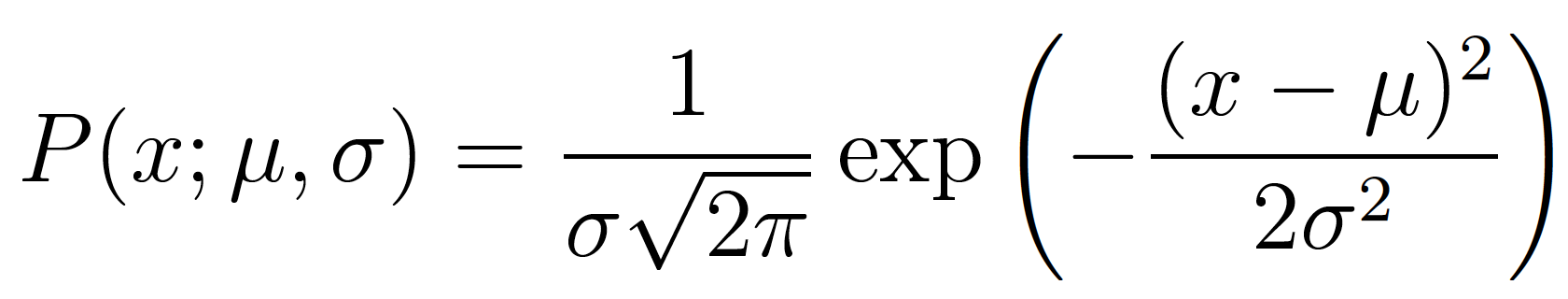P(x; μ, σ) 中的分号强调之后的符号代表概率分布的参数

在我们的例子中，观测到3个数据点的全（联合）概率为：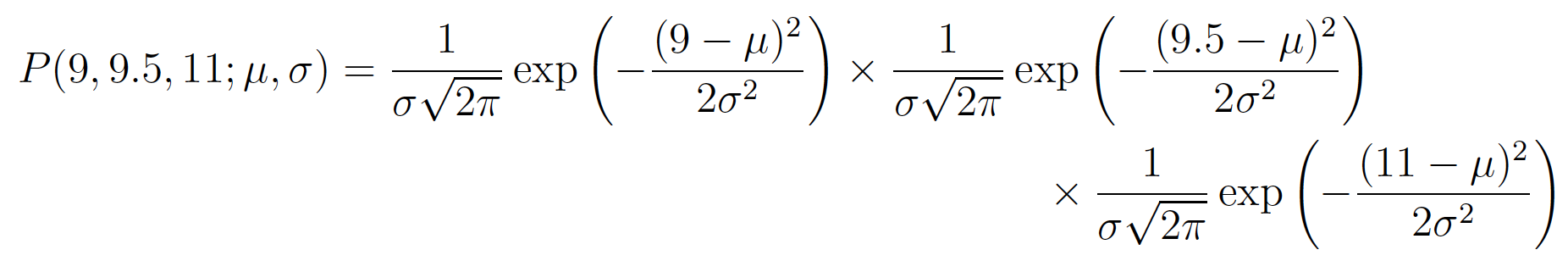我们只需找出能最大化以上表达式的值的μ和σ的值。

我们可以通过微分找到函数的最大（最小）值。我们只需找到函数的导数，将导数设为零，重新整理等式，即可得到值。

对数似然

实际上，对上面的全概率表达式求导很麻烦。所以我们基本上总是通过取自然对数对其加以简化。由于自然对数是单调递增函数，所以这么做绝对没问题。单调递增函数意味着随着x轴的值增加，y轴的值也同样增加（见下图）。这很重要，因为这确保了当概率的对数达到最大值时，原概率函数同样达到最大值。因此我们可以操作简化了的对数似然，而不是原本的似然。

为什么要取log

乘法变成加法，从而减少了计算量；同时，如果概率中含有指数项，如高斯分布，能把指数项也化为求和形式，进一步减少计算量；另外，在对联合概率求导时，和的形式会比积的形式更方便。

但其实可能更重要的一点是，因为概率值都在[0,1]之间，因此，概率的连乘将会变成一个很小的值，可能会引起浮点数下溢，尤其是当数据集很大的时候，联合概率会趋向于0，非常不利于之后的计算。

取对数不影响单调性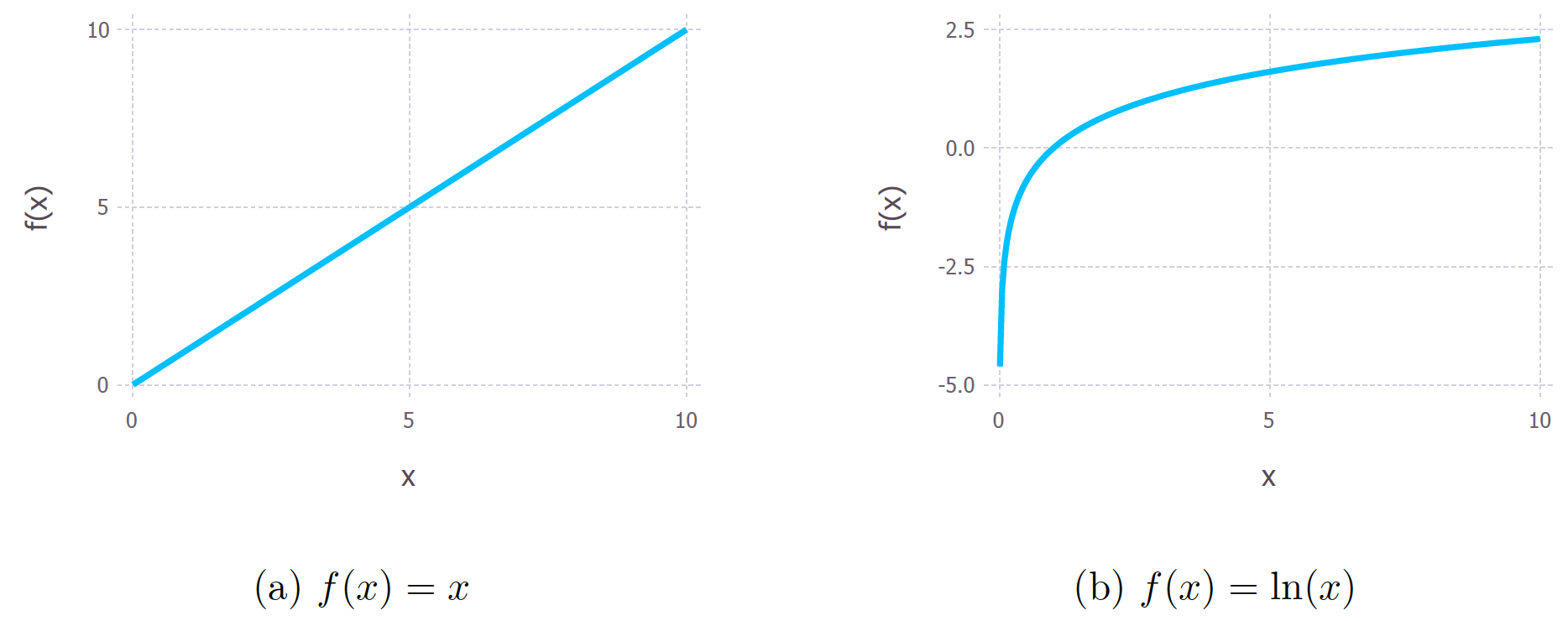因为相同的单调性，它确保了概率的最大对数值出现在与原始概率函数相同的点上。因此，可以用更简单的对数似然来代替原来的似然。

对原表达式取对数，我们得到：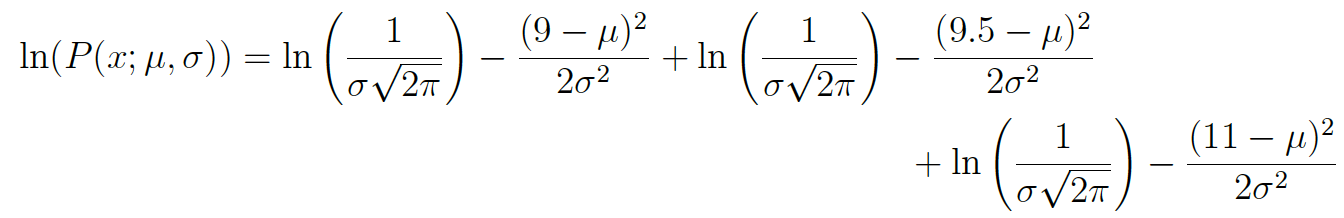据对数定律，上式可以简化为：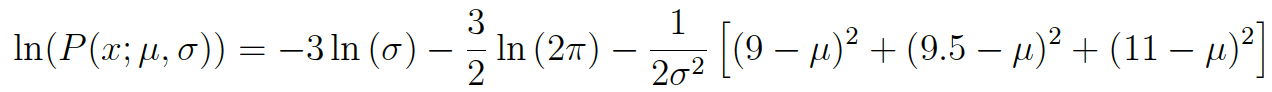对以上表达式求导以找到最大值。在这个例子中，我们将寻找均值μ的MLE。为此，我们求函数关于μ的偏导数：最后，我们将等式的左半部分设为0，据μ整理等式得到：这样我们就得到了μ的最大似然估计。同理，我们可以求得σ的最大似然估计

为什么是最大似然，而不是最大概率？

这只是统计学家在卖弄学问（不过他们的理由很充分）。大部分人倾向于混用概率和似然，但是统计学家和概率论学者区分了两者。以下等式突显了两者之所以容易混淆的原因：这两个表达式是相等的！所以这意味着什么？让我们先来定义P(data; μ, σ)。它的意思是“基于模型参数μ和σ观测到数据的概率”。值得注意的是，我们可以将其推广到任意数目的参数和任意分布。

另一方面，L(μ, σ; data)的意思是“我们已经观测到一组数据，参数μ和σ取特定值的似然”。

上面的等式意味着给定参数得到数据的概率等于给定数据得到参数的似然。然而，尽管两者相等，似然和概率根本上问的是不同的问题——一为数据，一为参数。这就是这一方法叫做最大似然而不是最大概率的原因。

二、贝叶斯推断

贝叶斯定理定义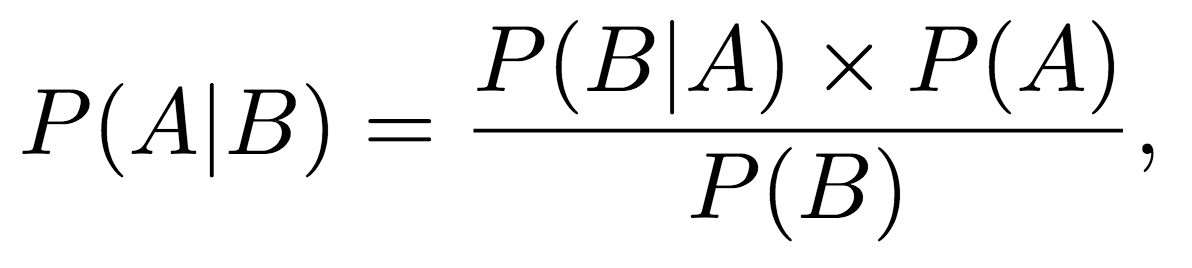贝叶斯定理如何允许我们纳入先验信念？

贝叶斯定理允许我们纳入先验信念，但是只看前文给出的等式，很难看出这是怎么办到的。所以让我们使用冰淇淋和天气的例子来说明。

A表示我们看到冰淇淋的事件，B表示天气事件。接着我们也许会问已知天气类型的前提下，销售冰淇淋的概率是多少？在数学上这写作P(A=冰淇淋销售 | B=天气类型)，对应于贝叶斯定理等式的左侧。

等式右侧的P(A)被称为先验。在我们的例子中，这是P(A=冰淇淋销售)，即不考虑天气类型的情况下，销售冰淇淋的（边缘）概率。P(A)被称为先验是因为我们可能已经知道冰淇淋销售的边缘概率。例如，我可能查看过数据，100个潜在顾客中，有30人在某处的某家店实际购买了冰淇淋。所以，在我知道任何关于天气的信息之前，P(A=冰淇淋销售) = 30/100 = 0.3。这样，贝叶斯定理让我们纳入了先验知识。

贝叶斯推断

首先，（在统计学上）推断是推理数据的种群分布或概率分布的性质的过程。上面说的最大似然其实就包含了这一过程。我们基于观察到的一组数据点决定均值的最大似然估计。

因此贝叶斯推断不过是使用贝叶斯定理推理数据的种群分布或概率分布的性质的过程。

将贝叶斯定理应用于分布

到目前为止，所有的例子中，贝叶斯定理的每一项的值都是单个数字。这意味着我们得到的答案也将是单个数字。然而，有时候单个数字可能不怎么合适。

在前文的冰淇淋的例子中，我们看到销售冰淇淋的先验概率是0.3。然而，如果0.3只是我的最佳猜测，我并不是非常确定，会怎么样？概率也可能是0.25或0.4。在这一情形下，用一个分布来表示我们的先验信念可能更加合适（见下图）。这一分布被称为先验分布。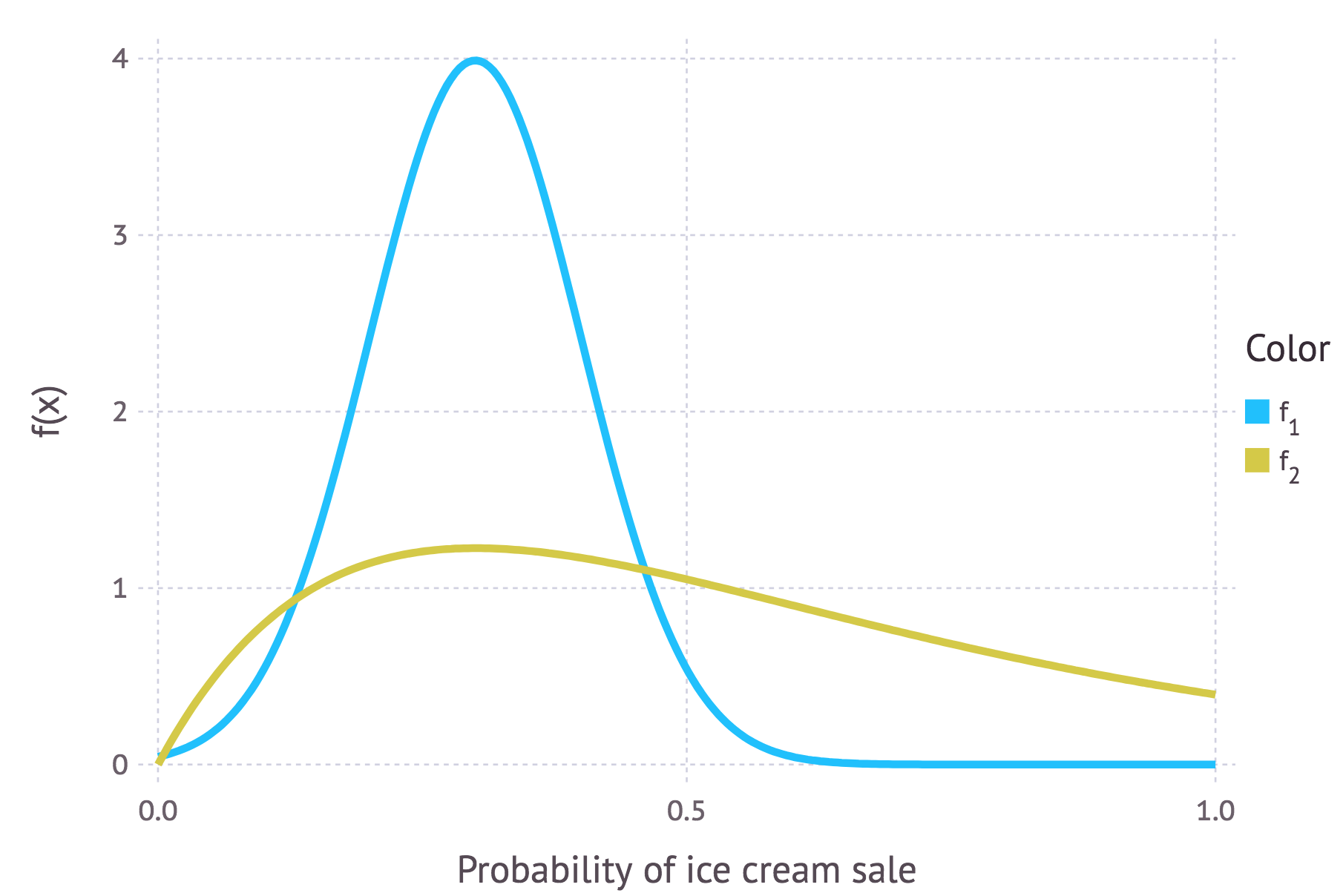上图为表示任意一天冰淇淋销售的两个分布。蓝色曲线和金色曲线的峰值均位于0.3附近，如前所述，这是我们对冰淇淋销售的先验概率的最佳猜测。而f(x)在其他处的值并不为零，表明我们并不是完全确信0.3是冰淇淋销售的真实值。蓝色曲线显示它可能是0到0.5之间的任何值，而金色曲线显示它可能是0和1之间的任何值。相比蓝色曲线，金色曲线更为舒展，峰值更低，这意味着金色曲线表达的先验概率“不那么确定”。

基于类似的方法，我们可以用分布表示贝叶斯定理中的其他项。当我们处理模型的时候，大多数情况下我们都需要使用分布。

贝叶斯定理的模型形式

前文介绍贝叶斯定理的定义时，我使用A、B表示事件。但关于贝叶斯定理的模型形式的文献往往使用不同的符号。

我们通常使用Θ而不是A。Θ表示一组参数。所以如果我们尝试估计高斯分布的参数值，那么Θ表示均值μ和标准差σ（在数学上写作Θ = {μ, σ}）。

我们通常使用data或者y = {y1, y2, ..., yn}，而不是B。这代表数据，也就是我们的观测集合。我会在等式中显式地使用data，希望这能让等式看起来不那么晦涩。

因此，贝叶斯定理的模型形式：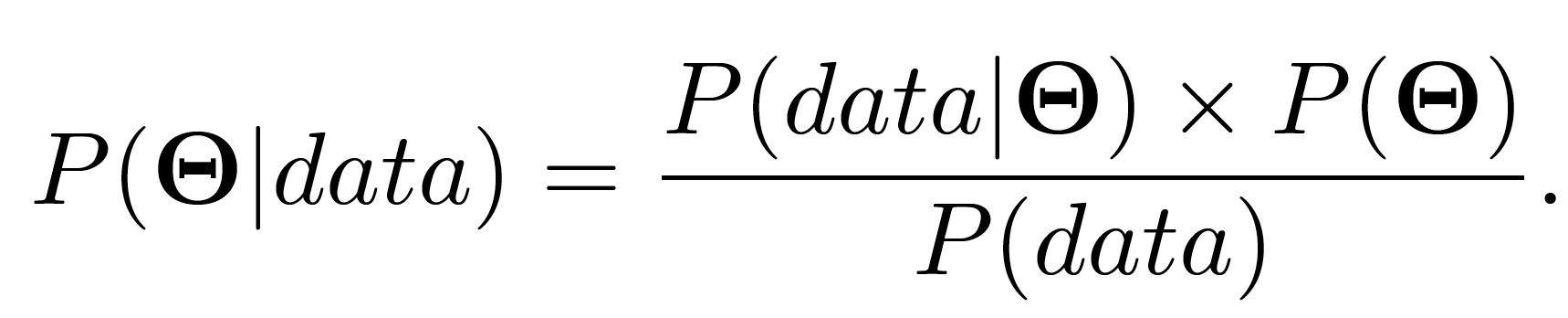P(Θ)为先验分布，表示我们关于参数的真值的信念，就像我们之前用分布表示我们关于冰淇淋销售的概率的信念。

等式左边的P(Θ|data)称为后验分布。它表示基于已经观测到的数据计算出等式右边的各项之后我们对参数的信念。

其实我们之间已经接触过P(data|Θ)。如果你读完了上面最大似然的内容，那么你会记得我们提到过L(data; μ, σ) 是（高斯分布的）似然分布。好，P(data|Θ) 正是这个，它是改头换面的似然分布。有时它写作ℒ(Θ; data)，都是一回事。有时它被称为证据。

因此，我们可以通过证据更新我们的先验信念来计算我们的参数的后验分布。

这给了我们充足的信息来讨论使用贝叶斯推断来推断参数的一个例子。但是首先……

为什么我完全忽视了P(data)？

除了数据的边缘概率之外，P(data)并没有什么特别的名字。记住，我们关心的是参数值，而P(data)并没有提到参数。事实上，P(data)甚至不是一个分布。它只是一个数字。我们已经观测到了数据，因此我们计算出P(data)。一般而言，结果我们发现计算P(data)会非常困难。

P(data)之所以重要，是因为它得出的数字是一个归一化常量。概率分布的一个必要条件是一个事件的所有可能性的概率之和为1（例如，投掷一枚6面骰得到1、2、3、4、5、6点的全概率等于1）。归一化常量通过确保分布之和（其实我应该说积分，因为通常我们碰到的是连续分布，不过目前这么说太过于卖弄学问了）等于1来确保所得的后验分布是真实概率分布。

在某些情况下，我们并不关心分布的这一性质。我们只关心分布的峰值何时出现，而不在乎分布是否归一化。在这一情况下，很多人把贝叶斯定理的模型形式写作其中∝表示“成比例”。这显式地表明了真实后验分布不等于右边的式子，因为我们没有考虑归一化常量P(data)。

共轭分布

你会注意到，我们所有关于分布的例子中都使用了高斯分布。主要的一个原因是这大大简化了数学。但是在贝叶斯推断的例子中 ，我们得计算两个分布的乘积。我说过这很凌乱，所以我没有详细列出数学计算过程。但是即便我自己没有进行这些数学计算，我早就知道后验分布会是高斯分布。因为高斯分布具有一个特别的性质，使得高斯分布易于处理。高斯分布和自身的高斯似然函数是共轭的。这意味着，如果我将一个高斯先验分布乘以一个高斯似然函数，我将得到一个高斯后验函数。后验与先验来自同一分布家族（它们都是高斯分布）意味着它们是共轭分布。在这个例子中，先验分布是一个共轭先验。

在很多推断的场景中，我们选择使所得分布共轭的似然和先验，因为这简化了数学。数据科学中的一个例子是隐含狄利克雷分布（LDA），这是一种在多个文档（语料库）中搜寻主题的无监督学习算法。

参考：

https://zhuanlan.zhihu.com/p/44520176

https://towardsdatascience.com/probability-concepts-explained-maximum-likelihood-estimation-c7b4342fdbb1

https://towardsdatascience.com/probability-concepts-explained-bayesian-inference-for-parameter-estimation-90e8930e5348

展开全文• 机器学习有三种典型的参数估计方法分别是极大似然估计(MLE)，极大后验估计(MAP)以及贝叶斯估计。除了参数估计，每种方法还对应有样本的密度估计。假设X=[xi]i=1nX=[x_i]_{i=1}^nX=[xi​]i=1n​。 MLE 极大似然是...
参数估计的三种方法
机器学习有三种典型的参数估计方法，分别是极大似然估计(MLE)，极大后验估计(MAP)以及贝叶斯估计。除了参数估计，每种方法还对应有样本的密度估计。假设$X=[x_i]_{i=1}^n$。
MLE
极大似然是通过下式估计参数。
$\theta^* = argmax_\theta P(X|\theta)$
该式可以这样理解，在给定参数$\theta$的情况下，求解具体概率$P(X|\theta)$的最大值，使得满足预期样本分布。上式往往通过下述方法求解：
$\theta^* = argmax_\theta P(X|\theta)=argmax_\theta \prod_{i=1}^nP(x_i|\theta)$
MAP
极大后验则给定样本的后验和参数的先验，求解下式：
$\theta^* = argmax_\theta P(\theta|X)$
可以这样理解，在给定观测样本集的条件下，估计最有可能的$\theta^*$。该式往往利用Bayes公式求解，由于：
$P(\theta|X) = \frac{P(X|\theta)P(\theta)}{P(X)}$
所以：
$\theta^* = argmax_\theta P(\theta|X) = argmax_\theta \frac{P(X|\theta)P(\theta)}{P(X)} = argmax_\theta \prod_{i=1}^n P(x_i|\theta)P(\theta)$
其中，$P(\theta)$为参数的先验。
贝叶斯估计
MLE和MAP方法有着共同点，即他们都是估计最可能的$\theta$，并极大化上述式子求解$\theta$，这被称为点估计。而Bayes估计承认明显的事实，参数$\theta$可能有多个与数据兼容的值，用Bayes公式计算在数据X上参数$\theta$的概率分布。
$P(\theta|X) = \frac{P(X|\theta)P(\theta)}{P(X)} = \frac{\prod_{i=1}^n P(x_i|\theta)P(\theta)}{P(X)}$
注意，尽管和MAP形式上一致，但贝叶斯估计无极大化过程。且有：
$P(\theta|X)P(X) = P(X|\theta)P(\theta)$
$\int P(\theta|X)P(X) d\theta = \int P(X|\theta)P(\theta) d\theta = P(X)$
密度估计
样本的密度估计即估算预测分布。即计算下式：
$P(x^*|X) = \int P(x^*|\theta)P(\theta|X)d\theta$
可以这样理解，$P(x^*|\theta)$是一个给定值$\theta$的预测。所以，积分可以当做由不同参数$\theta​$确定的预测的加权和，也就是期望。
由于MLE和MAP这两种参数估计方法均属于点估计，因此这两者在进行预测时
$P(x^*|X) = \int P(x^*|\theta)\delta(\theta-\theta^*)d\theta$
其中$\delta(z)$积分为1，并且在除了z=0以外的任何点都为0。这是因为，在点估计方式下，隐含的假设为：$\theta$只有一个可能的最优值$\theta^*$。因此这两种方法下，有：
$P(\theta^*|X) = \infty$
且$P(\theta \neq \theta^*|X) = 0$
由于$P(x^*|X) = \int P(x^*|\theta)\delta(\theta-\theta^*)d\theta = P(x^*|\theta^*)$。
因此，MLE和MAP的密度估计方法自然导出：给定$\theta^*$，预测样本的分布。
贝叶斯估计中却不是这种方法，这是因为贝叶斯估计给出的$P(\theta|X)$是概率分布，非极大化的结果。所以，无法用$\delta$函数对$P(\theta|X)$进行替换。
举例-一元正态分布
假设n个数据点$[x_i]_i=1^n$从均值方差均为1的正态分布中采样得到。本例的目的是利用这些观测数据重新估算参数$\mu, \sigma^2$。
MLE
利用极大似然估计，我们有
$P(X|\mu, \sigma^2) = \prod_{i=1}^n P(x_i|\mu, \sigma) = \prod_{i=1}^n Norm_{x_i}(\mu,\sigma)$
其中$Norm_{x_i}(\mu,\sigma) = \frac{1}{\sqrt{2\pi \sigma}}e^{-\frac{(x_i-\mu)^2}{\sigma^2}}$为点$x_i$的概率密度。
极大似然估计即：
$\mu^*,\sigma^{2*} = argmax_{\mu, \sigma^2} P(X|\mu,\sigma) = argmax_{\mu, \sigma^2} log P(X|\mu,\sigma^2) ​$
对$P(X|\mu,\sigma^2)$取对数，然后对$\mu,\sigma^2$分别求偏导为0可得最优解。
MAP
利用极大后验估计，我们有
$P(\mu,\sigma^2|X) = \frac{P(X|\mu,\sigma^2)P(\mu,\sigma^2)}{P(X)}$
其中$P(X|\mu,\sigma^2)$和MLE中一致，$\mu,\sigma^2$的先验分布假设为正态逆伽马分布。表达式如下：
$P(\mu,\sigma^2)$
$= NormInvGamma_{\mu,\sigma^2}(\alpha,\beta,\gamma,\delta)$
$= \frac{\sqrt{\gamma}}{\sigma\sqrt{2\pi}} \frac{\beta^\alpha}{\Gamma(\alpha)}(\frac{1}{\sigma^2})^{\alpha+1} e^{-\frac{2\beta+\gamma(\delta-\mu)^2}{2\sigma^2}}$
正态逆伽马分布是正态分布的共轭，它有4个参数,分别是$\alpha,\beta,\gamma,\delta$。共轭性具有以下特点：
任意分布与其共轭分布乘积的结果正比于一个具有新参数的共轭分布。我们以正态分布和正态逆伽马分布为例：
$Norm_{x_i}(\mu,\sigma^2)*NormInvGamma_{\mu,\sigma^2}(\alpha,\beta,\gamma,\delta) = \chi(x_i, \alpha,\beta,\gamma,\delta) * NormInvGamma_{\mu,\sigma^2}(\hat{\alpha}, \hat{\beta}, \hat{\gamma}, \hat{\delta})$
这在贝叶斯估计中很有用，具体地我们会在下一小节讲述。
回到正题，现在我们得到了$P(\mu,\sigma^2|X)$，对其直接进行极大化，通过对参数求偏导为0可求解得到最优解。
$\mu^*,\sigma^{2*}= argmax_{\mu,\sigma}P(\mu,\sigma^2|X) = argmax_{\mu,\sigma}log P(\mu,\sigma^2|X)$
贝叶斯估计
贝叶斯估计则要复杂一些，它和MAP具有同样的形式，不同的是在最后一步。
$P(\mu,\sigma^2|X)​$
$= \frac{P(X|\mu,\sigma^2)P(\mu,\sigma^2)}{P(X)}​$
$= \frac{\prod_{i=1}^n Norm_{x_i}(\mu,\sigma^2)NormInvGamma_{\mu,\sigma^2}(\alpha,\beta,\gamma,\delta)}{P(X)}​$
$= \frac{NormInvGamma_{\mu,\sigma^2}(\hat{\alpha}, \hat{\beta}, \hat{\gamma}, \hat{\delta})\prod_{i=1}^n \chi(x_i, \alpha,\beta,\gamma,\delta) }{P(X)}​$
由于后验$P(\mu,\sigma^2|X)$必须是有效的概率分布且和为1，而$NormInvGamma_{\mu,\sigma^2}(\hat{\alpha}, \hat{\beta}, \hat{\gamma}, \hat{\delta})$也是一个概率分布且和为1。因此有：
$P(\mu,\sigma^2|X)=NormInvGamma_{\mu,\sigma^2}(\hat{\alpha}, \hat{\beta}, \hat{\gamma}, \hat{\delta})$
其中，$\hat{\alpha} = \alpha+n/2, \hat{\gamma}=\gamma+n, \hat{\delta} = \frac{\gamma\delta+\sum x_i}{\gamma+n}, \hat{\beta} = 0.5\sum x_i^2 + \beta+0.5\gamma\delta^2-\frac{(\gamma\delta+\sum x_i)^2}{2(\gamma+n)}$
可以看到，利用贝叶斯公式我们可以得到参数的概率分布，这里我们看到使用共轭先验进行贝叶斯估计的第一个优点，比起MLE和MAP，这种方法得到的结果是一个closed-solution。并且，参数概率分布的峰值即为MAP的解。
当数据比较多的时候，参数被指定好，概率分布比较集中，此时把所有概率量放到MAP估计处是对后验的一个好的逼近。然而，当数据稀少的时候，许多可能的参数也许已经解释了数据并且后验很宽，这种情况下用点估计方法是不合适的。
密度估计
对于MLE和MAP，由于它们是点估计。可以分别直接采用$P(x^*|\mu^*,\sigma^{2*})$和$P(x^*|\mu^*,\sigma^{2*})P(\mu^*,\sigma^{2*})$预测新样本$x^*$的密度。
对于贝叶斯估计方法，有：
$P(x^*|X)$
$= \int \int P(x^*|\mu,\sigma^2)P(\mu,\sigma^2|X)d\mu d\sigma$
$=\int\int Norm_{x^*}(\mu,\sigma^2)NormInvGamma_{\mu,\sigma^2}(\hat{\alpha}, \hat{\beta}, \hat{\gamma}, \hat{\delta})d\mu d\sigma$
$=\chi(x^*,\hat{\alpha}, \hat{\beta}, \hat{\gamma}, \hat{\delta}) \int \int NormInvGamma_{\mu,\sigma^2}(\overline{\alpha}, \overline{\beta}, \overline{\gamma}, \overline{\delta})d\mu d\sigma$
显然，积分项为1，所以有：
$P(x^*|X) =\chi(x^*,\hat{\alpha}, \hat{\beta}, \hat{\gamma}, \hat{\delta})=\frac{1}{\sqrt{2\pi}}\frac{\sqrt{\hat{\gamma}}}{\sqrt{\overline{\gamma}}}\frac{\hat{\beta^{\hat{\alpha}}}}{\overline{\beta}^{\overline{\alpha}}}\frac{\Gamma(\overline{\alpha})}{\Gamma(\hat{\alpha})}$
其中，
$\overline{\alpha} = \hat{\alpha}+n/2, \overline{\gamma}=\hat{\gamma}+n, \overline{\delta} = \frac{\hat{\gamma}\hat{\delta}+\sum x_i}{\hat{\gamma}+n}, \overline{\beta} = 0.5\sum x_i^2 +\hat{ \beta}+0.5\hat{\gamma}\hat{\delta}^2-\frac{(\hat{\gamma}\hat{\delta}+\sum x_i)^2}{2(\hat{\gamma}+n)}$。
这里，我们可以看到使用共轭先验进行贝叶斯估计的第二个优点，它意味着积分是可以计算的，所以可以得到密度预测的一个不错的闭式表达式。
对大量数据而言，贝叶斯估计和MAP估计得到的样本密度是非常接近的。但是在样本量少的情况下，贝叶斯估计的分布更加平稳，这也意味着它更不确定样本密度的真实性。


展开全文MAP
• MCMC方法的目的是获得服从高维分布的样本，理论涉及平稳分布马尔科夫链转移概率等，还是比较麻烦且不好懂的，但好在网上已有不少讲解得比较详细的。对于统计计算而言，获得高维分布样本后可以用于计算高维空间的积分...
• 参数估计方法都是用已知的概率分布函数与拟合数据，然后估计出概率分布的参数。但是有时候数据的概率分布函数未知或者概率分布函数不能很好的拟合数据，这个时候就可以用非参数估计数据的概率密度函数。 非参数估计 ...
• 这是模式分类中的课程代码， ...对不同维数下的高斯概率密度模型，用最大似然估计方法对其参数进行估计，学习和掌握最 大似然估计方法。 (a) 编写程序，对表格2 中的类1 w 中的3 个特征i x ，分别求解最大似然估计μ
• 对于参数估计，统计学界的两个学派分别提供了不同的解决方法。 频率主义学派（Frequentist）认为参数虽然未知，但却是客观存在的固定值， 因此，可通过优化似然函数等准则来确定参数值； 贝叶斯学派（Bayesian）...
• 在本文中，提出了通过对频率零附近的累积归一化周期图应用拟合优度检验来估计潜在的长期依赖时间序列的存储参数方法。 大量模拟研究的结果表明，与传统的频域估计器相比，这种新的估计器性能良好，后者是基于...
• 参数估计方法有多种，这里我们分析三种基于概率的方法分别是最大似然估计（Maximum Likelihood）、贝叶斯估计（Bayes）和最大后验估计（Maximum a posteriori）。我们假设我们观察的变量是x，观察的变量取值...最大似然 贝叶斯
• 这里面都需要解决通信信号制式的自动识别和参数估计。另外， 信噪比（SNR）是接收信号的一个关键参数，它对通信系统的性能有着重要影响， 对它的估计也十分必要。直接扩频序列信号（DSSS）由于具有极低的功率谱密 度...
• 估计主要有两种方法分别是估计和极大似然估计，具体原理可参考统计学教材。 矩估计需要解n元方程，在R语言中有： 解一次方程：uniroot(),调用格式为; uniroot(f,interval,...,lower = min(interval), ...
• 解决这一问题的方法参数估计和非参数估计。非参数估计包含核密度估计。 离散型随机变量 概率函数：就是用函数的形式来表达概率； 概率分布： 概率分布函数（简称分布函数）：就是概率函数取值的累加结果，所以它...python
• 方法二是在实测光谱数据中注入独立同分布的随机噪声, 利用基于偏差函数最小化方法的混合优化算法多次进行反演计算, 将每次反演得到的薄膜光学参数估计值的统计均值作为薄膜真实光学参数的估计值, 以减小甚至消除随机...
• 一般来说，就这两个参数，再加上PV的时间分布，即一天的多少小时内产生这些PV，就可以估计网站的并发数了。计算公式如下： PC=PV/T*C*t 其中，PC是并发数，T是观测时间，即产生PV的时间长度，比如一天中的1
• 论文研究-正态型位置参数Bayes估计中基于P值的验前分布可信度建模.pdf, 基于Bayes方法完成对战技指标的分析，首要和关键的问题是选择合理可信的验前分布.以正态分布位置...
• ## 区间估计

千次阅读 2018-03-17 15:01:31
一.实验目的 分别使用Excel、SPSS和Python软件做区间估计，探究哪...在实际中，度量一个点估计的精度最直观的方法是给出未知参数的一个区间。1. 区间估计的概念设 是总体的一个参数， 是样本，在得到样本观测值后...
• 极大似然估计(Maximum Likelihood Estimator) 和最大后验估计(Maximum a posteriori Estimator)是机器学习概率模型中常用到的两种参数估计方法。两者分别来自频率学派和贝叶斯学派。两者差别在哪？MLE在规模大数据集...
• 今天主要复习一下两种参数估计的统计学方法分别是极大似然估计（MLE）和最大后验概率估计（MAP）。 问题背景 MLE MAP MLE与MAP之间的区别与联系 问题背景 以掷硬币为例。现在我们一共抛掷了10次硬币，其结果为...
•  极大似然法估计是以观测值出现的概率为最大作为估计准则的，它是一种觉的参数估计方法。 设是连续随机变量，其分布密度为，含有个未知参数。把个独立观测值分别代入中的，则得 将所得的个函数相乘，得 ...极大似然法
• 参数估计方法有多种，这里我们分析三种基于概率的方法分别是最大似然估计（Maximum Likelihood）、贝叶斯估计（Bayes）和最大后验估计（Maximum a posteriori）。我们假设我们观察的变量是x，观察的变量取值...最大似然 贝叶斯
• 我在非参数技术——Parzen窗估计方法文章和非参数估计-Parzen窗口函数法文章里面整理出了算法基本过程：利用第一篇博客给出的样本数据对给定的数据进行分类。分类的方法就是根据公式分别求出对于三个类的数值。公式...
• MLE与MAP分别对应两种学派的参数估计方法，频率派和贝叶斯派。频率派认为参数是未知的常量，而样本是随机变量，可以通过样本的概率分布估计参数的值。贝叶斯派认为参数是随机变量，其符合某种潜在先验概率分布prior...先验概率和后验概率
• 在上一篇博客中，我简单复习了矩估计，这篇博客将对点估计中的另外两个常见估计方法进行总结，分别是极大似然估计与最小二乘法，首先会对各自知识点进行归纳，最后论证这两种参数估计方法在特定条件下的转换关系。...机器学习 最小二乘法
• 相应的参数估计方法在MATLAB中都有现成的函数，比如aryule、arburg以及arcov等。 4.3.3 AR模型阶次的选择及实验设计 文献中介绍了五种不同的AR模型定阶准则，分别为矩阵奇异值分解（Singular Value ...matlab
• 软件可靠性建模是一个重要的研究领域，现有的软件可靠性模型基本上是...用该方法分别估计了5个实际软件系统的指数软件可靠性模型以及对数泊松执行时间模型，实验结果表明：该方法参数估计的精度高，对模型的适应性强。
• 目录随机信号的参数建模法三种模型MA模型AR模型ARMA模型AR模型参数估计matlab实例 随机信号的参数建模法 为随机信号建立参数模型是研究随机信号的一种基本方法，其含义是认为随机信号x（n）是由白噪声w（n） 激励......